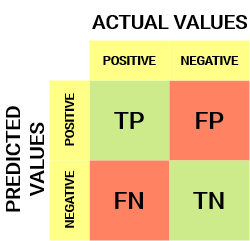# What is the confusion matrix?

Confusion matrix

A confusion matrix is a table that gives information on actual data and predicted data.

A Confusion matrix is an N x N matrix used for evaluating the performance of a classification model, where N is the number of target classes. The matrix compares the actual target values with those predicted by the machine learning model. This gives us a holistic view of how well our classification model is performing and what kinds of errors it is making.

For a binary classification problem, we would have a 2 x 2 matrix as shown below with 4 values:True Positive (TP)

• The predicted value matches the actual value
• The actual value was positive and the model predicted a positive value

True Negative (TN)

• The predicted value matches the actual value
• The actual value was negative and the model predicted a negative value

False Positive (FP) – Type 1 error

• The predicted value was falsely predicted
• The actual value was negative but the model predicted a positive value
• Also known as the Type 1 error

False Negative (FN) – Type 2 error

• The predicted value was falsely predicted
• The actual value was positive but the model predicted a negative value
• Also known as the Type 2 error